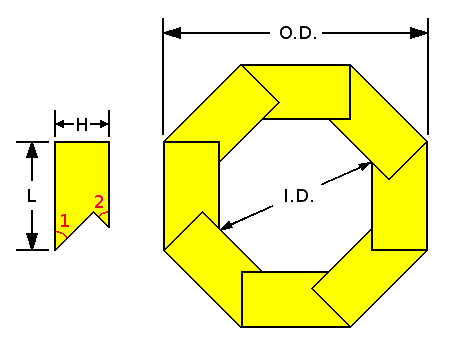# Calculators for Determining Birds Mouth Spar Stave Dimensions

In WoodenBoat No., 255, Peter Cassidy writes about the construction of a wooden spinnaker pole. To determine the dimensions of the staves that make up this pole, Peter consulted two online calculators provided by Duckworks Boatbuilders Supply. Duckworks has generously allowed us to reproduce those calculators here.

### Calculating the Basic Stave Dimensions: Given N, O.D. and I.D., calculate L, H and K (scroll down to view a list of all terms)

 Inputs N: O.D.: I.D.:
 Results L: H: A: K: M:

Status:

### Calculating the Taper of the Staves: Given N, O.D. and H, calculate L, I.D. and K

 Inputs N: O.D.: H:
 Results L: I.D.: A: K: M:

Status:### Definitions of the Terms Used in these Calculators

• O.D. is the outside diameter
• I.D. is the largest inside diameter
• 1 is the first angle of the stave
• 2 is the second angle of the stave
• L is the width of material measured on the outside
• H is the thickness of material
• N is the number of sides, or staves

#### Important Ratios

• K is the thickness to width ratio of staves or (H / L)
• M is the “conversion factor” or (O.D. / L)
• A is the inside to outside diameter ratio or (I.D. / O.D.)

#### Hints to avoid error messages (in the Status box)

• Input values must be numbers higher than zero (no negatives, no letters)
• The number of sides must be 5 or more
• I.D. must be smaller than O.D. (obviously)
• H or K must not be too small or too large (less obvious)
• H must be smaller than the radius or half the diameter